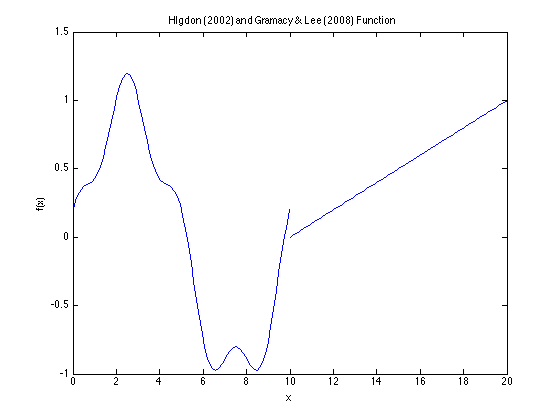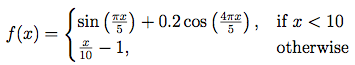## Higdon (2002) and Gramacy & Lee (2008) Function### Description:

Dimensions: 1

This function is a simple one-dimensional example used for illustrating methods of modeling computer experiment output. There is generally a random term ε ~ N(0, 0.12) added to the response.

### Input Domain:

The function is evaluated on x ∈ [0, 20].

### Modifications and Alternative Forms:

In its original form in Higdon (2002), the function consists only of the continuous sinusoidal portion on
x < 10, without the linear region. The cosine term is a sine term instead.

Gramacy et al. (2004) include the linear term, but it is on (35.75, 60] instead of [10, 20].

### References:

Gramacy, R. B., & Lee, H. K. (2008). Bayesian treed Gaussian process models with an application to computer modeling. Journal of the American Statistical Association, 103(483).

Gramacy, R. B., & Lee, H. K. (2009). Adaptive design and analysis of supercomputer experiments. Technometrics, 51(2).

Gramacy, R. B., Lee, H. K., & Macready, W. G. (2004, July). Parameter space exploration with Gaussian process trees. In Proceedings of the twenty-first international conference on Machine learning (p. 45). ACM.

Higdon, D. (2002). Space and space-time modeling using process convolutions. In Quantitative methods for current environmental issues (pp. 37-56). Springer London.